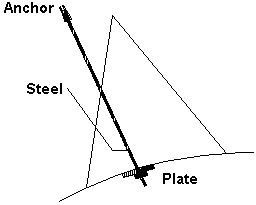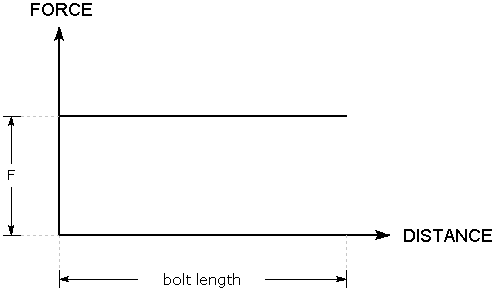The Rocscience International Conference 2021 Proceedings are now available. Read Now

Mechanically Anchored Bolts

The effective tensile capacity of a Mechanically Anchored bolt is dictated by the weakest of the following components:

• Anchor Capacity
• Steel tensile capacity

Therefore, when you enter these parameters in the Bolt Properties dialog, only the lowest valued parameter will be used in the analysis. The other two values will not be used.Mechanically anchored bolt

Bolt Force Diagram

When a Mechanically Anchored bolt intersects a wedge plane, it does not matter where the wedge plane intersects the bolt. The same force will be applied to the wedge, regardless of the point of intersection along the length of the bolt.

F = min ( f1, f2, f3 )

where f1, f2, f3 are the anchor, steel and plate capacities.

The Force Diagram for a Mechanically Anchored bolt is simply a horizontal line, representing a constant value of applied force, regardless of where a wedge plane intersects the bolt.Tensile bolt force diagram for mechanically anchored bolt